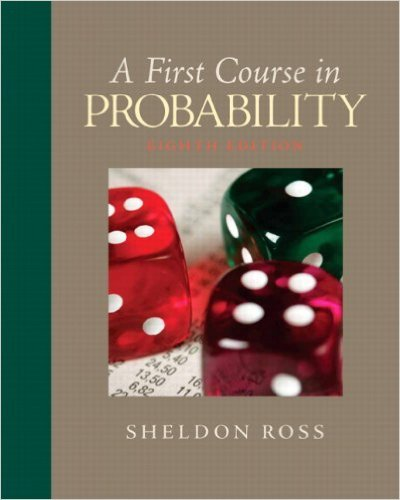×

×

Textbooks / Statistics / First Course in Probability 8

# First Course in Probability 8th Edition - Solutions by Chapter## Full solutions for First Course in Probability | 8th Edition

ISBN: 9780136033134First Course in Probability | 8th Edition - Solutions by Chapter

Solutions by Chapter
4 5 0 289 Reviews
##### ISBN: 9780136033134

This textbook survival guide was created for the textbook: First Course in Probability, edition: 8. Since problems from 10 chapters in First Course in Probability have been answered, more than 25486 students have viewed full step-by-step answer. The full step-by-step solution to problem in First Course in Probability were answered by , our top Statistics solution expert on 11/23/17, 05:06AM. This expansive textbook survival guide covers the following chapters: 10. First Course in Probability was written by and is associated to the ISBN: 9780136033134.

Key Statistics Terms and definitions covered in this textbook
• Alternative hypothesis

In statistical hypothesis testing, this is a hypothesis other than the one that is being tested. The alternative hypothesis contains feasible conditions, whereas the null hypothesis speciies conditions that are under test

• Average run length, or ARL

The average number of samples taken in a process monitoring or inspection scheme until the scheme signals that the process is operating at a level different from the level in which it began.

• Biased estimator

Unbiased estimator.

• Bimodal distribution.

A distribution with two modes

• C chart

An attribute control chart that plots the total number of defects per unit in a subgroup. Similar to a defects-per-unit or U chart.

• Central composite design (CCD)

A second-order response surface design in k variables consisting of a two-level factorial, 2k axial runs, and one or more center points. The two-level factorial portion of a CCD can be a fractional factorial design when k is large. The CCD is the most widely used design for itting a second-order model.

• Central tendency

The tendency of data to cluster around some value. Central tendency is usually expressed by a measure of location such as the mean, median, or mode.

• Chi-square test

Any test of signiicance based on the chi-square distribution. The most common chi-square tests are (1) testing hypotheses about the variance or standard deviation of a normal distribution and (2) testing goodness of it of a theoretical distribution to sample data

• Combination.

A subset selected without replacement from a set used to determine the number of outcomes in events and sample spaces.

• Completely randomized design (or experiment)

A type of experimental design in which the treatments or design factors are assigned to the experimental units in a random manner. In designed experiments, a completely randomized design results from running all of the treatment combinations in random order.

• Continuous distribution

A probability distribution for a continuous random variable.

• Correlation coeficient

A dimensionless measure of the linear association between two variables, usually lying in the interval from ?1 to +1, with zero indicating the absence of correlation (but not necessarily the independence of the two variables).

• Correlation matrix

A square matrix that contains the correlations among a set of random variables, say, XX X 1 2 k , ,…, . The main diagonal elements of the matrix are unity and the off-diagonal elements rij are the correlations between Xi and Xj .

• Designed experiment

An experiment in which the tests are planned in advance and the plans usually incorporate statistical models. See Experiment

• Distribution function

Another name for a cumulative distribution function.

• Enumerative study

A study in which a sample from a population is used to make inference to the population. See Analytic study

• Error mean square

The error sum of squares divided by its number of degrees of freedom.

• Error sum of squares

In analysis of variance, this is the portion of total variability that is due to the random component in the data. It is usually based on replication of observations at certain treatment combinations in the experiment. It is sometimes called the residual sum of squares, although this is really a better term to use only when the sum of squares is based on the remnants of a model-itting process and not on replication.

• Forward selection

A method of variable selection in regression, where variables are inserted one at a time into the model until no other variables that contribute signiicantly to the model can be found.

• Geometric mean.

The geometric mean of a set of n positive data values is the nth root of the product of the data values; that is, g x i n i n = ( ) = / w 1 1 .